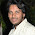## Sunday, 3 February 2013

### C PROGRAM: TO CALCULATE ARITHMETIC MEAN OF N NUMBERS

CODING:
#include<stdio.h>
#include<conio.h>
void main( )
{
int n;
float a,i,am,sum=0;

clrscr( );
printf("Enter number of terms ");
scanf("%d",&n);
printf("Enter %d values ",n);
for(i=1;i<=n;i++)
{
scanf("%f",&a[i]);
sum=sum+a[i];
}
am=sum/n;
printf("\n\nArithmetic Mean = %f",am);
getch( );
}CODINGOUTPUT

For any query or suggestion please comment below.....

1.2.1.